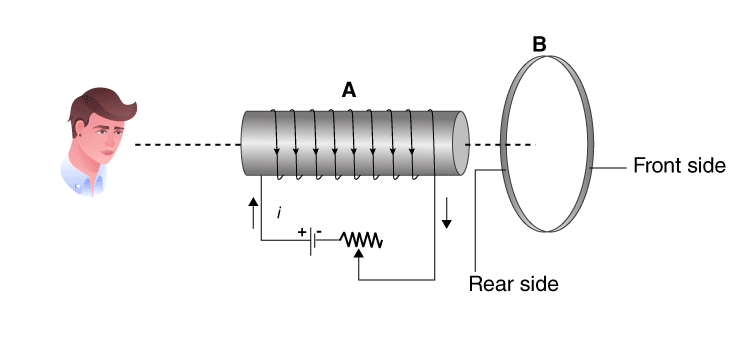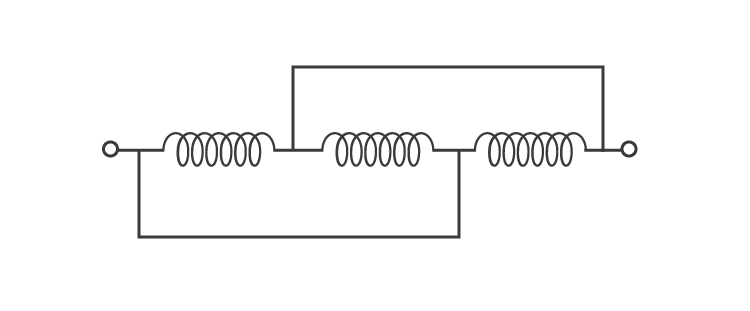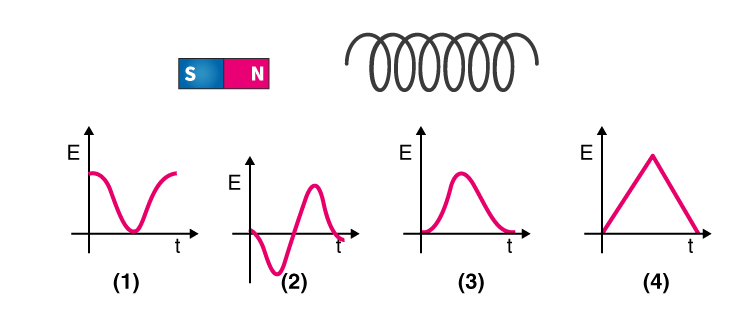# Electromagnetic Induction and Alternating Currents MCQ for NEET

NEET  Physics is the scoring paper in the medical entrance examination. Here, you will discover the NEET Physics MCQ Questions for all Concepts as per the latest syllabus. Practice more on a regular basis with these NEET Physics objective questions on air pollution and improve your subject knowledge & problem-solving skills along with time management. NEET Physics Electromagnetic Induction and Alternating Currents Multiple Choice Questions make you feel confident in answering the question in the exam & increases your scores to high.

## MCQ on Electromagnetic Induction and Alternating Currents

1. How to increase the energy stored in an inductor by four times?
(a) By doubling the current

(b) This is not possible
(c) By doubling the inductance
(d) By making current √2times

Answer: (a) By doubling the current

2. Consider an inductor whose linear dimensions are tripled and the total number of turns per unit length is kept constant, what happens to the self-inductance?
(a) 9 times
(b) 3 times
(c) 27 times
(d) $$\frac{1}{3}$$ times

3. Lenz law is based on which of the following conservation
(a) Charge
(b) Mass
(c) Momentum
(d) Energy

4. What will be the acceleration of the falling bar magnet which passes through the ring such that the ring is held horizontally and the bar magnet is dropped along the axis of the ring?
(a) It depends on the diameter of the ring and the length of the magnet
(b) It is equal due to gravity
(c) It is less than due to gravity
(d) It is more than due to gravity

Answer: (c) It is less than due to gravity

5. An observer is seeing the setup of an aluminium ring B facing an electromagnet A. The current I through A can be altered if(a) B is independent of an increase or decrease of I
(b) If I increases, A will attract B
(c) If I increase, A will repel B
(d) If I decrease, A will repel B

Answer: (c) If I increase, A will repel B

6. What is the equivalent inductance of the following circuit if the pure inductance is 3.0H(a) 9H
(b) 3H
(c) 1H
(d) 2H

7. What is the need for laminating the core of a transformer?
(a) To reduce the resistance in the winding
(b) To reduce the eddy currents
(c) To reduce the hysteresis
(d) None of the above

Answer: (b) To reduce the eddy currents

8. Which of the following represent the variation of induced EMF with respect to time t if a short bar magnet is moved along its axis and has a constant velocity.9. A wire loop is rotated in a magnetic field such that the frequency of change of direction of the induced emf is
(a) Six times per revolution
(b) Four times per revolution
(c) Twice per revolution
(d) Once per revolution

10. Which of the following is the equivalent quantity of mass in electricity?
(a) Current
(b) Charge
(c) Potential
(d) Inductance

11. What is the unit of inductance?
(a) Volt/ampere
(b) Joule/ampere
(c) Volt-ampere/sec
(d) Volt-sec/ampere